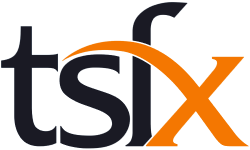# Further Mathematics Course Catalogue

Further Mathematics consists of two areas of study, a compulsory Core area of study to be completed in Unit 3 and an Applications area of study to be completed in Unit 4. The Core comprises ‘Data analysis’ and ‘Recursion and financial modelling’. The Applications comprises two modules to be completed in their entirety, from a selection of four possible modules: ‘Matrices’, ‘Networks and decision mathematics’, ‘Geometry and measurement’ and ‘Graphs and relations’. ‘Data analysis’ comprises 40 per cent of the content to be covered, ‘Recursion and financial modelling’ comprises 20 per cent of the content to be covered, and each selected module comprises 20 per cent of the content to be covered. Assumed knowledge and skills for the Core are contained in the General Mathematics Units 1 and 2 topics: ‘Computation and practical arithmetic’, ‘Investigating and comparing data distributions’, ‘Investigating relationships between two numerical variables’, ‘Linear graphs and modelling’, ‘Linear relations and equations’, and ‘Number patterns and recursion’. For each module there are related topics in General Mathematics Units 1 and 2.

In undertaking these units, students are expected to be able to apply techniques, routines and processes involving rational and real arithmetic, sets, lists and tables, diagrams and geometric constructions, algebraic manipulation, equations, and graphs. They should have a facility with relevant mental and by-hand approaches to estimation and computation. The use of numerical, graphical, geometric, symbolic, financial and statistical functionality of technology for teaching and learning mathematics, for working mathematically, and in related assessment, is to be incorporated throughout each unit as applicable.

## Course List

To filter the course list, enter your search text into the below field

For example, entering Unit 3 into the search field would show all Unit 3 Further Mathematics Courses.
\Learnworlds\Codeneurons\Pages\ZoneRenderers\CourseCards